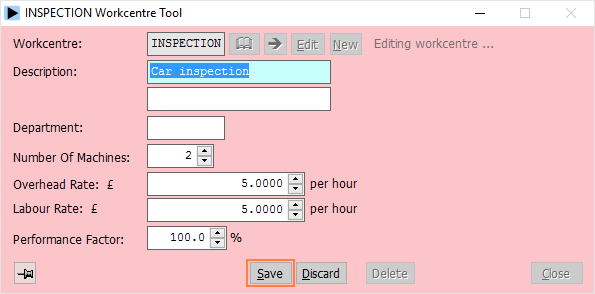The various machines and areas on your shopfloor should be divided into workcentres. These workcentres are entered into Herschel using the workcentre tool:The following information is held for each workcentre:

 Workcentre Code The workcentre’s code. Description The description of the workcentre. Department The department to which the workcentre belongs. Number Of Machines The number of machines in the workcentre. This affects the amount of available hours the workcentre has free each day. For example, if you have an 8 hour working day and there are four machines in a workcentre, the system will assume that the workcentre can do a total of 32 hours of work each day. Overhead Rate The overhead rate per hour. This is the cost per hour of the overheads (e.g. heat, light) involved when running the workcentre. Labour Rate The labour rate per hour. This is the cost per hour of the labour (e.g. wages) involved when running the workcentre. Performance Factor The performance factor of the workcentre. If this is 100% the system will assume the workcentre will take one hour to do work that is expected to take one hour. If the performance factor is, say 110%, the system would assume that the workcentre is working 10% faster than expected, and that work that normally taking one hour to complete would only take 54.5 minutes. Likewise, a performance of say 90% would make the system assume that work would take longer than originally expected (66.7 minutes).

The overhead rate and labour rate are used by the transfer route costs options in the Assemblies module and for job costing purposes. If you do not intend using these options they can be omitted.